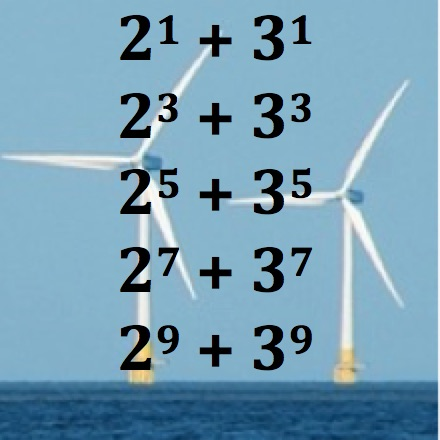What pattern can you see in the last digits of the powers of 2?

What about the last digits of the powers of 3?

What patterns can you see in the last digits for the numbers in the picture?

Can you explain why all these numbers are multiples of 5?

What about the 99th powers $$2^{99} + 3^{99}$$?

What can you find out about $$1^{99} + 2^{99} + 3^{99} + 4^{99}$$?

What other patterns with powers can you find that have special properties?